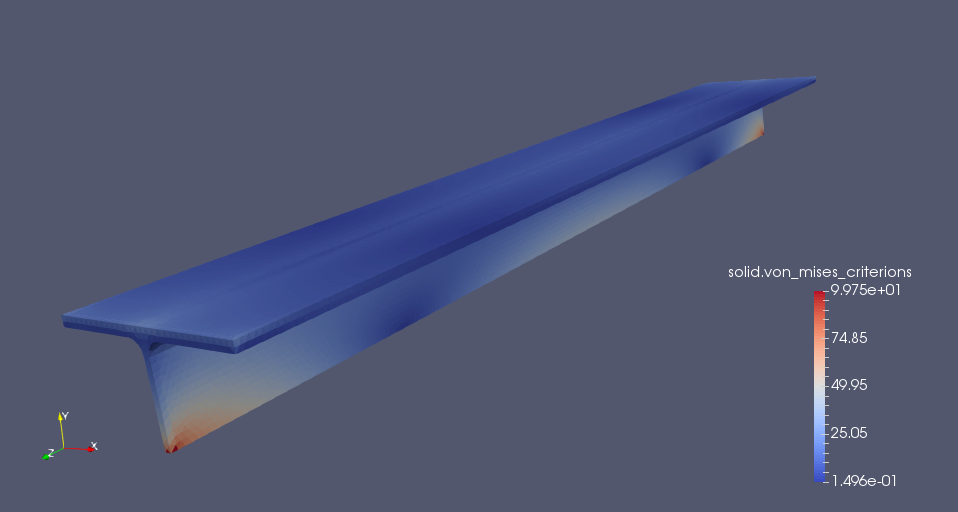# T-beam

## 1. Introduction

Tee Section, also known as T beam or T bar, is a structural beam with a “T” shaped cross section. Tee section is generally made of plain carbon steel. Manufacturing methods of “T” sections are hot rolling, extrusion and plate welding. T bars are often used for general fabrication.

## 2. Running the case

The command line to run this case is

``mpirun -np 4 feelpp_toolbox_solid --case "github:{repo:toolbox,path:examples/modules/csm/examples/t-beam}"``

## 3. Data files

The case data files are available in Github here

## 4. Model/Geometry

First, we need to build the model, which we are going to do using the Gmsh-software.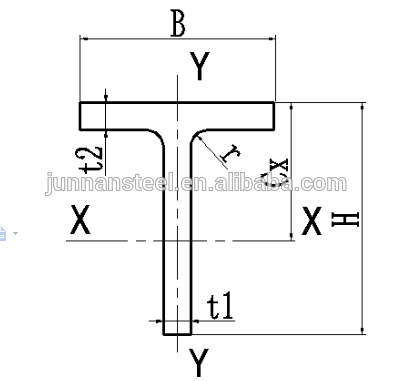In this case we will use the following data:

• h = 150 mm

• B = 300 mm

• t1 = 10 mm

• t2 = 15 mm

• r = 20 mm

• l = 2000 mm

The finished geometry and the meshed model with 10 mm mesh-size: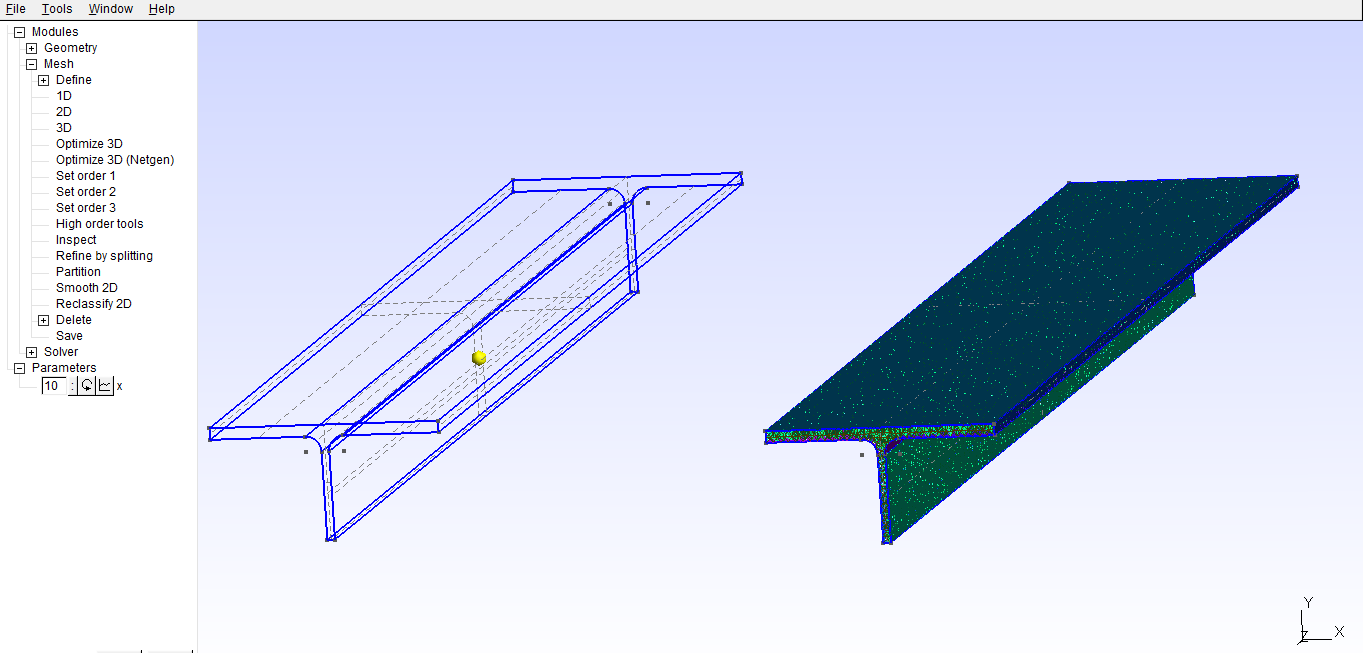## 5. Materials and boundary conditions

### 5.1. Materials

The beam is made of steel, so we need to use the following data:

• E = 210 GPa

• nu = 0.3

• rho = 7870 kg/m3

### 5.2. Boundary conditions

Both ends of the beam are fixed and the force is applied to the upper surface, in the center of the beam. In this case we will apply 50000 N force to the upper surface (named „load”).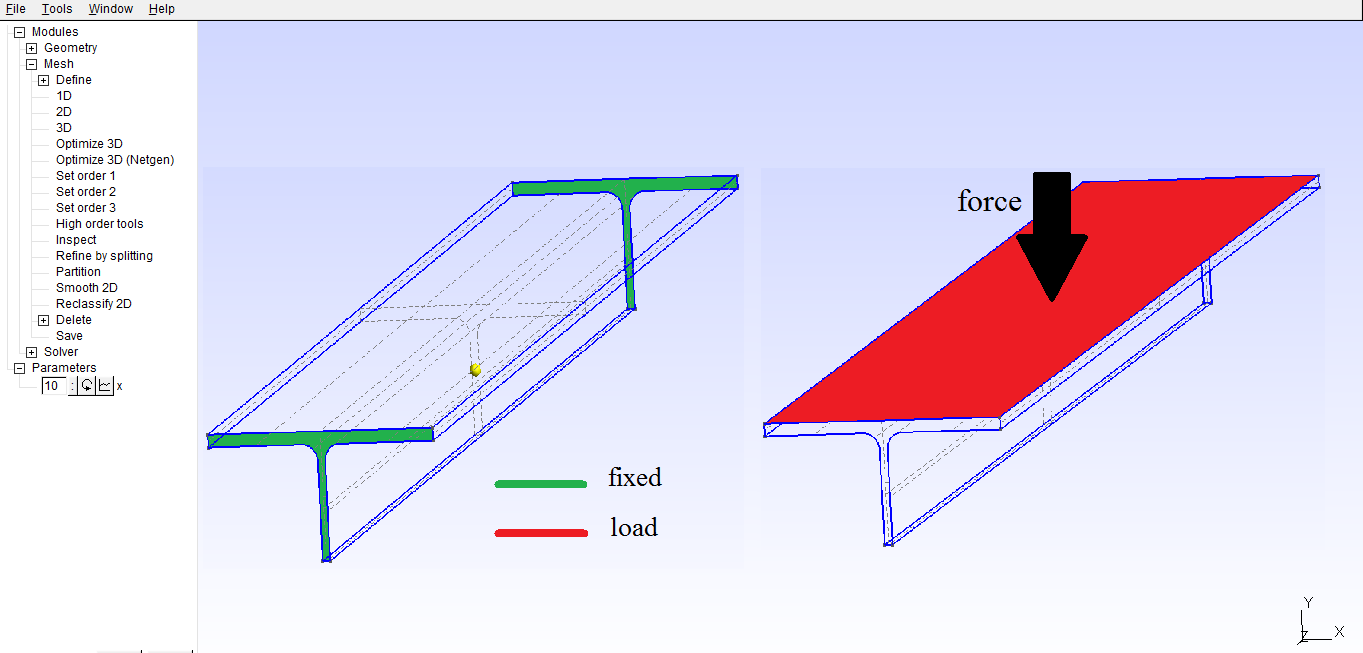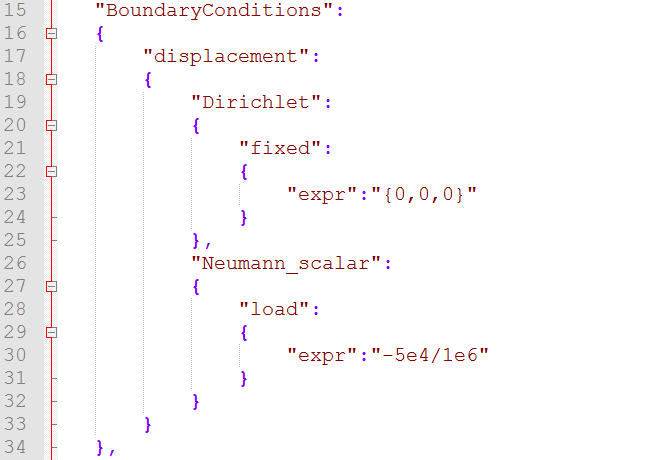## 6. Results

### 6.1. Displacement

Maximum displacement: 0.4854 mm

 In the lower picture a 100x scale factor was used to make the displacement easy to see.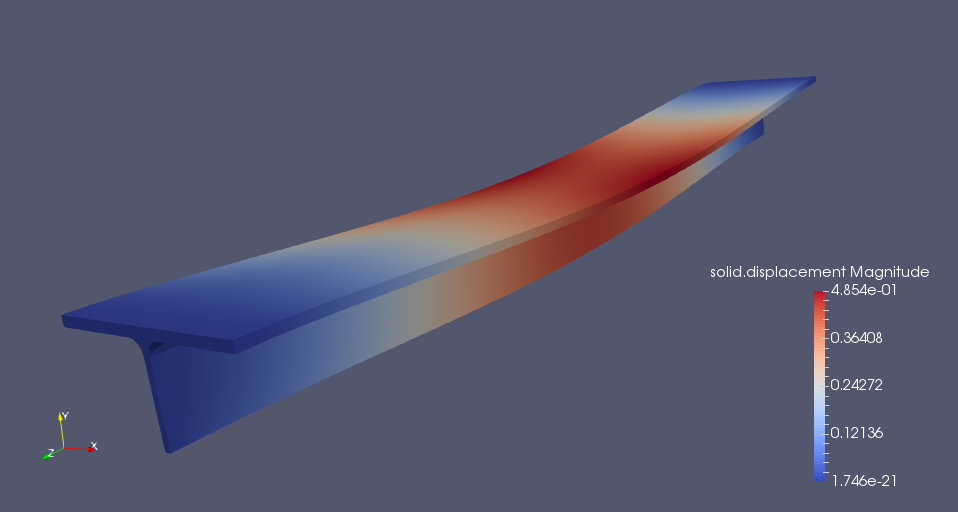### 6.2. Von-Mises Criterions

Maximum stress: 99,75 MPa